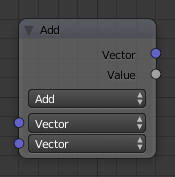# Vector Math Node¶Vector Math Node.

The Vector Math node performs the selected math operation on vectors. Select the math function by clicking the up-down selector where the “Add” selection is shown.

## Inputs¶

Vector
Input vector 1 (upper). The value can be provided by another node or set manually.
Vector
Input vector 2 (lower). The value can be provided by another node or set manually.

## Properties¶

Operation

Select the math function for conversion.

Hint

\begin{align}\begin{aligned}Vector &= input_1 + input_2\\Value &= \frac{abs(Vector_x) + abs(Vector_y) + abs(Vector_z)} {3.0}\end{aligned}\end{align}
Subtract

Subtracting input 1 and 2.

Hint

\begin{align}\begin{aligned}Vector &= input_1 - input_2\\Value &= \frac{abs(Vector_x) + abs(Vector_y) + abs(Vector_z)} {3.0}\end{aligned}\end{align}
Average

Averaging input 1 and 2.

Hint

\begin{align}\begin{aligned}Vector &= \frac{input_1+input_2} {|input_1+input_2|}\\Value &= |input_1+input_2|\end{aligned}\end{align}
Dot Product

Algebraic operation that takes two equal-length sequences of vectors 1 and 2 and returns a single number. The Result is a scalar.

Hint

$Value = input_1 . input_2$
Cross Product

Geometric binary operation on two vectors 1 and 2 in three-dimensional space. It results in a vector which is perpendicular to both and therefore normal to the plane containing them. The Result is a vector.

Hint

\begin{align}\begin{aligned}Vector &= \frac{input_1 \times input_2} {|input_1 \times input_2|}\\Value &= |input_1 \times input_2|\end{aligned}\end{align}
Normalize

Normalizing input 1.

Hint

\begin{align}\begin{aligned}Vector &= \frac{input_1} {|input_1|}\\Value &= |input_1|\end{aligned}\end{align}

## Outputs¶

Vector
Output vector, converted by the node.
Value
Output value, converted by the node.